# IoT applied to AirZone¶

Internet Of Thing (IoT) is the capacity of the electronic that surrounds us to interact and make our life easier.

The UniPlot use case presented below will give an extensive example of how to use UniPlot’s feature in this framework while navigating through.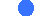cURL requestsREST APISQL DataBaseServices in UniPlot

Note

The whole code and template for the use case is available in the sample directory `UniPlot\Samples\IoT`

• AirZone1.ic: the code
• AirZone.ipz: the template

## 1. Why Controlling an A/C system with UniPlot¶

In this example there is a flat where the AirZone system is installed to allow the user to control how the Air Conditionning will heat-up or cool each individual room.

### 1.1 The system¶

A representation of this system is shown below. Each output of the conducts are equipped with an electrical motor controlled grid that is managed by the AirZone system based on the analysis of each room temperature.

The main machine blows air at a given temperature, and each grid will open or close itself to regulate the temperature of the rooms.

Indeed each room is equipped with a thermostat where a specific target temperature can be chosen for the room.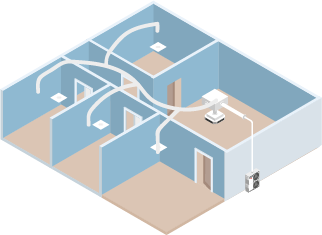This system is meant to be working on its own but it does not provide any history of the system’s state over the time.

### 1.2 UniPlot and AirZone¶

AirZone provides the user with the possibility to interact with the system thanks to a RESTful API.

UniPlot can then interact with this API to:Get data from it (temperature, set point, ON/OFF…) using the POST method:

POST http://XXX.XXX.XXX.XX:3000/api/v1/hvac With the following body:
```{
"systemID": n (system number),
"zoneID": m (zone number)
}
```Send temperature set-points via an home-made user interface using the PUT method:

PUT http://XXX.XXX.XXX.XX:3000/api/v1/hvac With the following body:
```{
"systemID": n (system number),
"zoneID": m (zone number),
"parameter" (parameter to modify, e.g "setpoint"): f (value)
}
```

As we can get data from the API, we will store it in an SQL database to be able to plot all the data over a period of time and do statistics and system analysis.

## 2. Discuss with the API: `AirZone_curl_POST()`¶

### 2.1 The cURL POST method¶

First things first, we need to contact the API to get some information.

UniPlot comes with the cURL library that will help us create our request.

```// Initialize the request
curl = curl_easy_init();
o = [. cnt = 0, data = [.]];
// Preparing options
oOptions = [.
URL =  "http://" + IPCASA + ":3000/api/v1/hvac",
COPYPOSTFIELDS = "{\"systemID\":0,\"zoneID\":" + ltostr(key) + "}",
HTTPHEADER = ["charset: utf-8"; "Accept: application/json"; "Content-Type: application/json"],
CURLOPT_WRITEDATA = o];
// Setting options
curl_easy_setopt(curl, oOptions);
// Send the request
r = curl_easy_perform(curl);
```

As one can see above, creating a request is done in 3 steps.Initialize the request

```curl = curl_easy_init();
```Preparing the options

```oOptions = [.
URL =  "http://" + IPCASA + ":3000/api/v1/hvac",
COPYPOSTFIELDS = "{\"systemID\":0,\"zoneID\":" + ltostr(key) + "}",
HTTPHEADER = ["charset: utf-8"; "Accept: application/json"; "Content-Type: application/json"],
CURLOPT_WRITEDATA = o];
```

This is the trickiest part where all the important information goes. Each line is a flag that corresponds to a specific action in our request. Some of them will always be present and some will depend on what you need to achieve. All the flags are explained here: https://curl.se/libcurl/c/curl_easy_setopt.html

• `URL`: the URL where the request is sent. It will always be present
• `COPYPOSTFIELDS`: This is the body needed in the request which holds the information of the system we want to know the status of.
• `HTTPHEADER`: It gives a description of what we send (here JSON encoded in utf-8).
• `WRITEFUNCTION`: It is a call back function used to store the answer received from the API.
• `CURLOPT_WRITEDATA`: It tells the previous callback function what to use to store the data ( here the object o).Linking the options to the curl request:

```curl_easy_setopt(curl, oOptions);
```Executing the request:

```r = curl_easy_perform(curl);
```

The following code will check whether an answer was received or not and then transform the information to use it in UniPlot

```if (r != 0) {
return curl_easy_strerror(r); // Return a possible error message
} else {
bool = curl_easy_cleanup(curl); // Needed to close the curl
s = "";
if (o.cnt == 0) {
return s;
}
for (i in 1:o.cnt) { // Unpack the data stored by the callback function
s = s + mem_unpack(o.data[i]);
}
s_txt = utf8_decode(s);
print(s_txt);
svDataName = AirZonesvDataName(); // Vector of human readable room names.

systemID.zoneID[key] = [.];
// We store the information in a UniScript object
for (ssName in svDataName) {
s_txt_pos = strfind(s_txt, "\"" + ssName + "\"");
if (s_txt_pos != 0) {
s_txt_cut = strextract(s_txt, s_txt_pos + strlen("\"" + ssName + "\"") + 1);
val = strtok(s_txt_cut, ",");
systemID.zoneID[key][ssName] = strtrim(strtok(s_txt_cut, ","));
}
}
}
```

The output of this function gives the following object to be used in UniPlot

```[.
= [.
zoneID = [.
 = [.
air_demand = "0",
coldStage = "1",
coldStages = "1",
errors = "[]",
floor_demand = "0",
heatStage = "1",
heatStages = "1",
humidity = "65",
maxTemp = "27",
minTemp = "15",
mode = "3",
name = "\"Salon\"",
on = "0",
roomTemp = "21.600000381469727",
setpoint = "22.5",
systemID = "1",
units = "0",
zoneID = "1",
],
 = [.
air_demand = "0",
coldStage = "1",
coldStages = "1",
errors = "[]",
floor_demand = "0",
heatStage = "1",
heatStages = "1",
humidity = "74",
maxTemp = "27",
minTemp = "15",
mode = "3",
name = "\"Hab.Matrimo\"",
on = "0",
roomTemp = "21",
setpoint = "18",
systemID = "1",
units = "0",
zoneID = "2",
],
```

## 3. Store the data: `AirZone_DB()`¶

Now that we can contact the API and get the information of our system, let’s store it in an sql database to decide later on what to do with it.We start by creating the database

```db = sqlite3_open(ssSrcDir + "/AirZone.db");
```We then create the tables needed if they do not exist

```for (zone in zones) {
sqlite3_execute(db, "create table if not exists " + zone ...
+ "(" + svValName + " varchar(100), " ...
+ svValName + " varchar(100), " ...
+ svValName + " varchar(100), " ...
+ svValName + " varchar(100), " ...
+ svValName + " varchar(100));");
}
b = sqlite3_execute(db, "create table if not exists TimeStamp(" ...
+ "TimeStamp varchar(100), " ...
+ "Year varchar(100), " ...
+ "Month varchar(100), "...
+ "Day varchar(100), ...
+ "YearMonthDay varchar(100));");
}
```

In this example we create 1 table per room and each table will hold 5 information:

• TimeStamp
• Room Temperature
• Room Temperature Setpoint
• Room Regulation state (ON/OFF)
• Room Air Demande (blowing or not)

Next to these tables we create another one to store date information under different form:

• TimeStamp
• Year
• Month
• Day
• YearMonthDayThen we call the function created earlier to get a set of fresh data and also inquire the actual time stamp

```Temperatures = AirZone_curl_POST();
t = DT_GetCurrentTime();
```Next step is to store the information in the tables

```for (zone in 1:len(zones)) {
obj = Temperatures.zoneID[zone];
sqlite3_execute(db, "insert into " + zones[zone] + " values("+ sprintf("%.4f", t) + "," ...
+ obj.roomTemp + "," ...
+ obj.setpoint + "," ...
+ obj.on + "," ...
+ obj.air_demand+ ");");
}
```And for the time stamps

```ssYear = DT_Format(t, "20%y");
ssMonth = DT_Format(t, "%m");
ssDay = DT_Format(t, "%d");
ssYearMonthDay = "'" + DT_Format(t, "20%y.%m.%d") + "'";
request = "insert into TimeStamp values("+ sprintf("%.4f", t) + "," + ssYear + "," + ssMonth + "," + ssDay + "," + ssYearMonthDay + ");";
sqlite3_execute(db, request);
```Finally we close properly the database

```db = sqlite3_close(db);
```

## 3. Let UniPlot work: `AirZone_DB_service_ON()`¶

We have created a structure able to call a REST API using curl library, fetch some information on our system and store it in a database.

We now want to gather data for a given period to be able to analyze how our system works.

To do so UniPlot can create a kind of service called Timer.

The function `AirZone_DB_service_ON()` is in charge of creating this timer for us

```def AirZone_DB_service_ON() {
_g().AirZone = [.];
_g().AirZone["Timer"] = AppNewTimer("AirZone_DB", AirZone_dtUpdate * 1000);
log_warning("uniscript", "AirZone", "AirZone Service ON (Refresh rate: " + ltostr(AirZone_dtUpdate) + "s)");
}
```

We use the global object `_g()` to store a dedicated object `AirZone` to which we assign the identifier of the timer

```_g().AirZone["Timer"] = AppNewTimer("AirZone_DB", AirZone_dtUpdate * 1000);
```

When this function is executed, the function AirZoneDB will be called every `AirZone_dtUpdate` seconds.

To Stop it we also created the following function which kills the timer by getting its identifier thank to the object created earlier

```def AirZone_DB_service_OFF() {
AppKillTimer(_g().AirZone["Timer"]);
log_warning("uniscript", "AirZone", "AirZone Service OFF");
}
```

## 4. Time to use the data¶

Now that we have setup our database and that it stores at a given frequency the state of the AC controller and the flat temperature, we want to make good use of this data and plot it.

In the following sections we will see a possible way to plot the data into an existing template via a small interface and how to use UniPlot features to live update the diagrams.

### 4.1 Plot it: `AirZone_FetchAndPlot(bWatch, DateMin, DateMax)`¶

This function will accept 3 parameters:

• `bWatch`: Use for continuous plotting. We will come back to it later on.
• `DateMin, DateMax`: It is the range of data we want to plot.

The data will be plotted in the following template.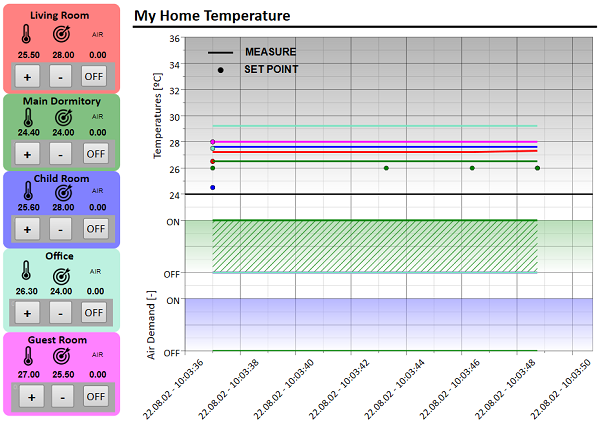To start we must open a template prepared to receive the data

```// Open the template
if (bWatch == 0) {
ssName = strcat(SplitPath(source(stack(0))), "");
ssSrcDir = sum(SplitPath(ssName)[1, 2]);
_g().Airzone = [. "ssSrcDir" = ssSrcDir, ...
"hPage" = hPage];
print(_g().Airzone);
} else {
ssSrcDir = _g().Airzone["ssSrcDir"];
hPage = _g().Airzone["hPage"];
}
```Then we gather the dataset handles to be updated and store it into an object to make the interaction easier. Each dataset has a name in the template. So we store the dataset handle in a key that has the dataset name.

```// Prepare data replacement
//hvPage = DocGetAllPages(hDoc, FALSE, FALSE);
hvData = PageGetAllDatasets(hPage);
o_Assignment = [.]
for (i in 1:len(hvData)) {
o_Assignment[ObjGetName(hvData[i])] = hvData[i];
}
```Time to interrogate the database to fetch the data.

Request creation We create the correct requests for each zone using SQL syntax

```"SELECT TimeStamp.TimeStamp, RoomTemp, SetPoint, AcOn, AirDemande "... // We select the columns to extract
+ "FROM TimeStamp "... // In the main TimeStamp table
+ "INNER JOIN " + zone + " " ... // Making an inner join with the table corresponding to the zone we analyze
+ "ON TimeStamp.TimeStamp = " + zone + ".TimeStamp " ... // using TimeStamp as index
+ " WHERE TimeStamp.YearMonthDay >= " + DateMin + " AND TimeStamp.YearMonthDay <= " + DateMax ... // only within the requested time frame
+ ";";
```

The code looks like this

```ssName = strcat(SplitPath(source( stack(0))), "");
db = sqlite3_open(ssSrcDir + "/AirZone.db");

o = [.];
for (zone in zones) {
if (bWatch == 0) {
request = "SELECT TimeStamp.TimeStamp, RoomTemp, SetPoint, AcOn, AirDemande "...
+ "FROM TimeStamp "...
+ "INNER JOIN " + zone + " " ...
+ "ON TimeStamp.TimeStamp = " + zone + ".TimeStamp " ...
+ " WHERE TimeStamp.YearMonthDay >= " + DateMin + " AND TimeStamp.YearMonthDay <= " + DateMax ...
+ ";";
} else {
request = "SELECT TimeStamp.TimeStamp, RoomTemp, SetPoint, AcOn, AirDemande "...
+ "FROM TimeStamp "...
+ "INNER JOIN " + zone + " " ...
+ "ON TimeStamp.TimeStamp = " + zone + ".TimeStamp " ...
+ "WHERE TimeStamp.TimeStamp=(SELECT max(TimeStamp.TimeStamp) FROM TimeStamp);";
}
}
```

Request execution We ask UniPlot to execute the request and store the output in `smTimeStamps`

```smTimeStamps = sqlite3_execute(db, request);
```

Replacing the data That data has to be assigned to its dataset.

As shown below, each dataset has been given a name which is the concatenation of the zone name (office, bedroom etc…) and the variable (Temperature, Set-point, …).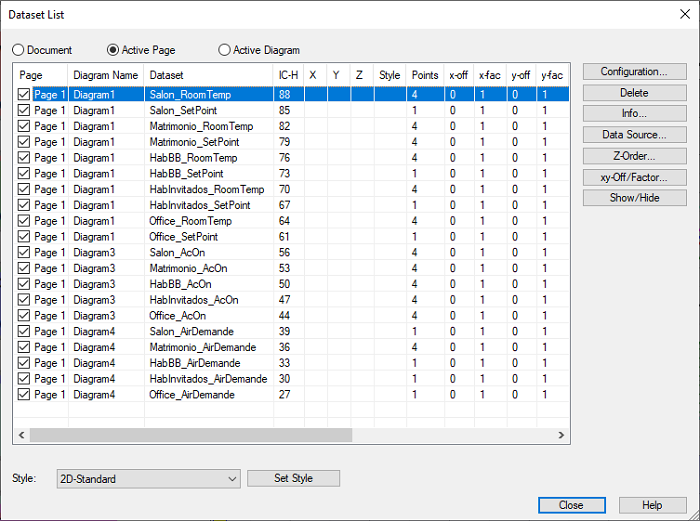The code will create an object whose keys are the name of the dataset and the values the handles

```for (idx in 2:len(svValName)) {
hData = o_Assignment[zone + "_" + svValName[idx]];
rvX = strtod(smTimeStamps[;1]);
rvY = strtod(smTimeStamps[;idx]);
if (bWatch == 1) {
rmXY = XYGetData(hData);
rvX = [rmXY[;1];rvX];
rvY = [rmXY[;2];rvY];
}
bool = XYSetData(hData, rvX, rvY);
}
```

Closing the database

```bool = sqlite3_close(db);
```

Updating the template

```PageReplot(hPage);
log_info("uniscript", "AirZone", "AirZone Plot update");
```

After all these efforts we should get the following output.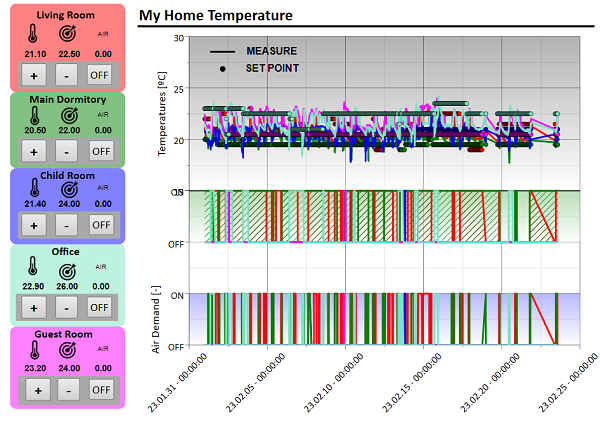### 4.2 Let’s make it user friendly: `AirZone_Interface()`¶

The `AirZone_FetchAndPlot(bWatch, DateMin, DateMax)` function mentionned in the previous chapter would benefit being called by an interface where the user can select start and end dates to be plotted. This is what the `AirZone_Interface()` function is about.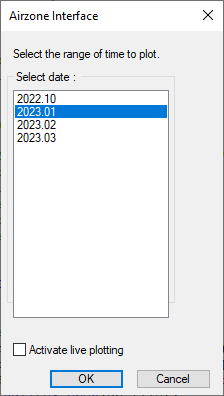The interface creation is built using the following philosophy. As one can see, it displays the months where data is available. To do so we need to interrogate the database get this information. The we format it properly and create the dialog template. Then the dialog is launched, the user select the period he wants to analyze. With this information, the `AirZone_FetchAndPlot` function is called.Let’s retrieve the database and store its identifier in db

```// Database
// Opening and creation if needed
ssName = strcat(SplitPath(source( stack(0))), "");
ssSrcDir = sum(SplitPath(ssName)[1, 2]);
db_file = ssSrcDir + "AirZone.db";
db = sqlite3_open(db_file);
```We then execute an SQL call onto `db` to retrieve all the time stamp information and close the database.

```smTimeStamps = sqlite3_execute(db, "select * from TimeStamp;");
bool = sqlite3_close(db);
```A bit of formating of the `smTimeStamps` string matrix lead us to have a usable ListBoxText usable in the init string of the dialog box.

```svYearMonth = ST_set(DT_Format(strtod(smTimeStamps[;1]), "20%y.%m"));
svYearMonth_List = CreateListBoxText(svYearMonth, 2);
svYearMonthDay = ST_set(DT_Format(strtod(smTimeStamps[;1]), "%x"));
svYearMonthDay_List = CreateListBoxText(svYearMonthDay, svYearMonthDay);
```To create our dialog box we will now prepare the init string and the template. If you have a doubt on how it works you can read the DialogBox documentation.

```// Initialization string
svInitString = ["Select date: ", svYearMonth_List, "0"]; //svYearMonthDay_List, "0"]
// Creation of template
svTemplate = ["Select the range of time to plot.", ...
"|G10                        |", ...
"|M10                          |", ...
strempty(1, 10), ...
"|BActivate live plotting|"];
```The dialog box ready, we launch it using the `DialogBox` function and store the output in `DateMin` and `DateMax`. Also notice the `bContinuousPlot` variable, I will mention it later on.

```// Launch DialogBox
svOutput = DialogBox(svTemplate, svInitString, "Airzone Interface");
if (svOutput == "DLG_CANCEL") {
return 0;
}
<svList, svSel> = GetListBoxText(svOutput);
DateMin = "'" + svList + ".01'";
DateMax = "'" + svList[len(svList)] + ".31'";
bContinousPlot = strtol(svOutput);
```Time to finally call our FetchAndPlot function. And the template will be populated with the desired data.

```AirZone_FetchAndPlot(0, DateMin, DateMax);
```### 4.3 Continuous Plotting: `FileWatchAdd()`¶

We now have two sources of data to analyze.Direct reading from the APIOur SQL database with our recordings

To do so we created a simple interface which aim is to show the user the data available and to plot it as seen in the previous chapter. In this earlier part I mentionned the `bContinuousPlot` which the user can set to 1 by clicking on the related checkbox. This check box will enable an automatic update of the template when new data is available.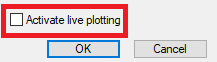If set to 1, in the `AirZone_Interface()` the `FileWatchAdd` function is called along with the callback function `AirZone_FetchAndPlot_WATCH()`.

```// Setting up continous plotting
if (bContinousPlot) {
}
```

The aim here is to tell UniPlot to check whenever the database is modified (so a new set of data has been stored) and to call the function `AirZone_FetchAndPlot_WATCH`. Which in its turn will call `AirZone_FetchAndPlot` whith the boolean `bWatch` set at 1.

```def AirZone_FetchAndPlot_WATCH(rsFile, bWatch) {
// This function has been created to support the FileWatch which has only 1 argument
AirZone_FetchAndPlot(bWatch);
}
```

We saw earlier that the `bWatch` variable changes the code behavior. As here we want to activate a continuous plotting, this variable has 2 effects :

• It retrieves the template actually in use which is stored in `_g().Airzone` to start updating it instead of creating a new one.
```ssSrcDir = _g().Airzone["ssSrcDir"];
hPage = _g().Airzone["hPage"];
```
• If modifies the SQL request to retrieve all the data available up to the actual time.
```request = "SELECT TimeStamp.TimeStamp, RoomTemp, SetPoint, AcOn, AirDemande "...
+ "FROM TimeStamp "...
+ "INNER JOIN " + zone + " " ...
+ "ON TimeStamp.TimeStamp = " + zone + ".TimeStamp " ...
+ "WHERE TimeStamp.TimeStamp=(SELECT max(TimeStamp.TimeStamp) FROM TimeStamp);"
```

The rest of the function is used as previously to plot the updated graph.

## 5. Control the A/C AirZone system with UniPlot¶

I mentionned in the UniPlot and AirZone chapter that the API offers two methods.

• POST to read info from the system
• PUT to send control request to the system.

Thanks to this I will now empower my template with controls (buttons) that can switch ON and OFF my A/C and even set the desired temperature!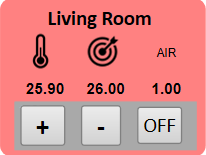To do so, each button of my template will be assigned a callback function and specific parameters depending on the need.

### 5.1 Adding the callback to our button¶

To add a callback function to a textbox, one has to right-click on it and select Properties in the context menu. Then go to the Properties tab. In this tab:

• Check Display as a button
• Check `OnClick`-Callback-Name
• Add a callback function name as follows `FunctioName?Param1,Param2,Param3`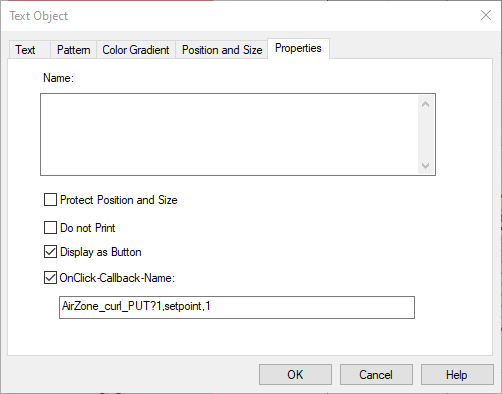```AirZone_curl_PUT?1,setpoint,1
```

The string placed in the callback function name will be parsed in the function afterward.

### 5.2 The callback function `AirZone_curl_PUT()`¶

`bool = AirZone_curl_PUT(_zoneID, _action, _val)`

Return Value

bool is TRUE (1) if no error happened during the process.

Parameters

`_zoneID`

_zoneID is the zone we want to act on.

`_action`

_action is the action that will be executed.

`_val`

_val is the value is for the action. For example the temperature setpoint.

The parameters can come from the UniScript call where they are explicitely given, or from the Callback function name as explained earlier.Parse the arguments

hTB is the handle of the textbox and with this parameter and the function `ObjectGetCallbackFunctionName` we get the name of the callback function that we parse using the `strtok` function. We then store only the parameters as a vector in `sv`.

```hTB = _g()._handle();
ssFuncName = ObjectGetCallbackFunctionName(hTB, "ObjectClickCallback");
sv = strtok(ssFuncName, "?");
// sv = FunctioName?Param1,Param2,Param3
// sv --> function names
// sv --> coma separated arguments
if (len(sv) == 2) {
sv = strtok(sv, ", ");
} else {
log_warning("uniscript", "Check parameter input for AirZone request -> function?zoneID,action,val");
return 0;
}
```Store the arguments

If the function is called without arguments we use what was coming from the `sv` variable, otherwise we use the arguments.

```if (nargsin() == 0) { // Sub Call
if (len(sv) == 3) {
zoneID = sv;
action = sv;
val = sv;
} else {
log_warning("uniscript", "Check parameter input for AirZone request -> ?zoneID,action,val");
return 0;
}
} else if (nargsin() == 3) {
zoneID = _zoneID;
action = _action;
val = _val;
}
```Setpoint preparation

If the user clicked the + or - action from our control, we need to know the actual temperature setpoint to add or remove a degree from it and later on send it to the A/C system. We also use the occasion to switch on the zone sending `AirZone_curl_PUT(zoneID, "on", "1")`.

```// Request preparation if action is temperature setpoint
if (action == "setpoint") {
setpoint = strtod(AirZone_curl_POST(strtol(zoneID), "setpoint"));
if (val == "1") val = setpoint + 1 else val = setpoint - 1;
val = ltostr(val);
AirZone_curl_PUT(zoneID, "on", "1")
}
```Sending the request

The following code will send the PUT request to the system with the correct zone, action and value to the system.

```// Curl request creation
curl = curl_easy_init();
data = "{\"systemID\":0,\"zoneID\":4}"
o = [. cnt = 0, data = [.]];
// Preparing options
oOptions = [.
URL =  "http://"+IPCASA+":3000/api/v1/hvac",
CUSTOMREQUEST = "PUT",
COPYPOSTFIELDS = "{\"systemID\":0,\"zoneID\":"+zoneID+",\"" + action + "\":" + val + "}",
HTTPHEADER = ["charset: utf-8";"Accept: application/json";"Content-Type: application/json"]];
// Setting options
curl_easy_setopt(curl, oOptions);
r = curl_easy_perform(curl);
//curl_easy_setopt(curl, CURLOPT_CUSTOMREQUEST, NULL) // To avoid further requests to be set as PUT
if (r != 0) {
return curl_easy_strerror(r);
} else {
bool = curl_easy_cleanup(curl);
// Setting up format
__FF_FieldUpdate(0, 0, 0, 0, FFU_ONDATACHANGE);
b=PageReplot(GetParent(GetParent(hTB)));
return 1;
}
```

### 5.3 Airzone field function¶

To close the loop, if you open the template AirZone.ipz and check one of the text field to display the temperature and its setpoint that I use a field function that uses everything we created up to know to display a numeric information on the state of our system.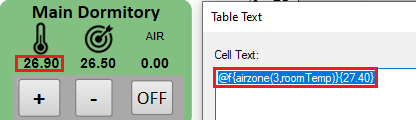The field function uses `AirZone_curl_POST` as defined previously and will be called everytime the page is refreshed.

```def __ff_airzone(hDoc, hPage, hLayer, hText, nAction, svParameter)
{
// Argument parsing
if (len(svParameter) == 2) {
zoneID = strtol(svParameter);
action = svParameter;
} else {
log_warning("uniscript", "Check parameter input for AirZone request -> ?zoneID,action");
return "??";
}
info = AirZone_curl_POST(zoneID, action);
return info;
}
```

## Conclusion¶

This use case covered a wide range of UniPlot functionality so that you can apply them to your own projects. We saw that UniPlot can be your new interface to your IoT at home, but also to monitor and control installations at your work place and it will of course give you all the tools you need to get insight on the data created.

id-1870160